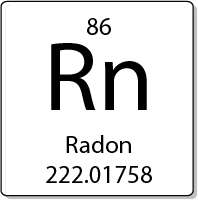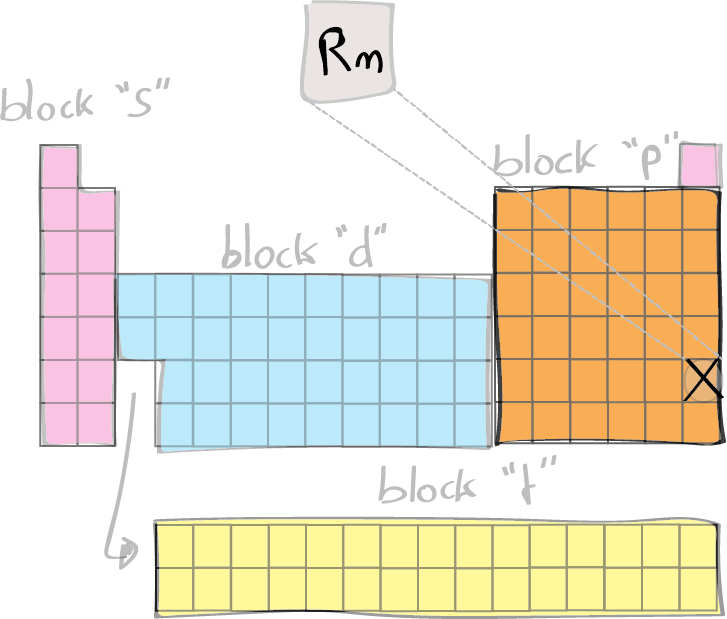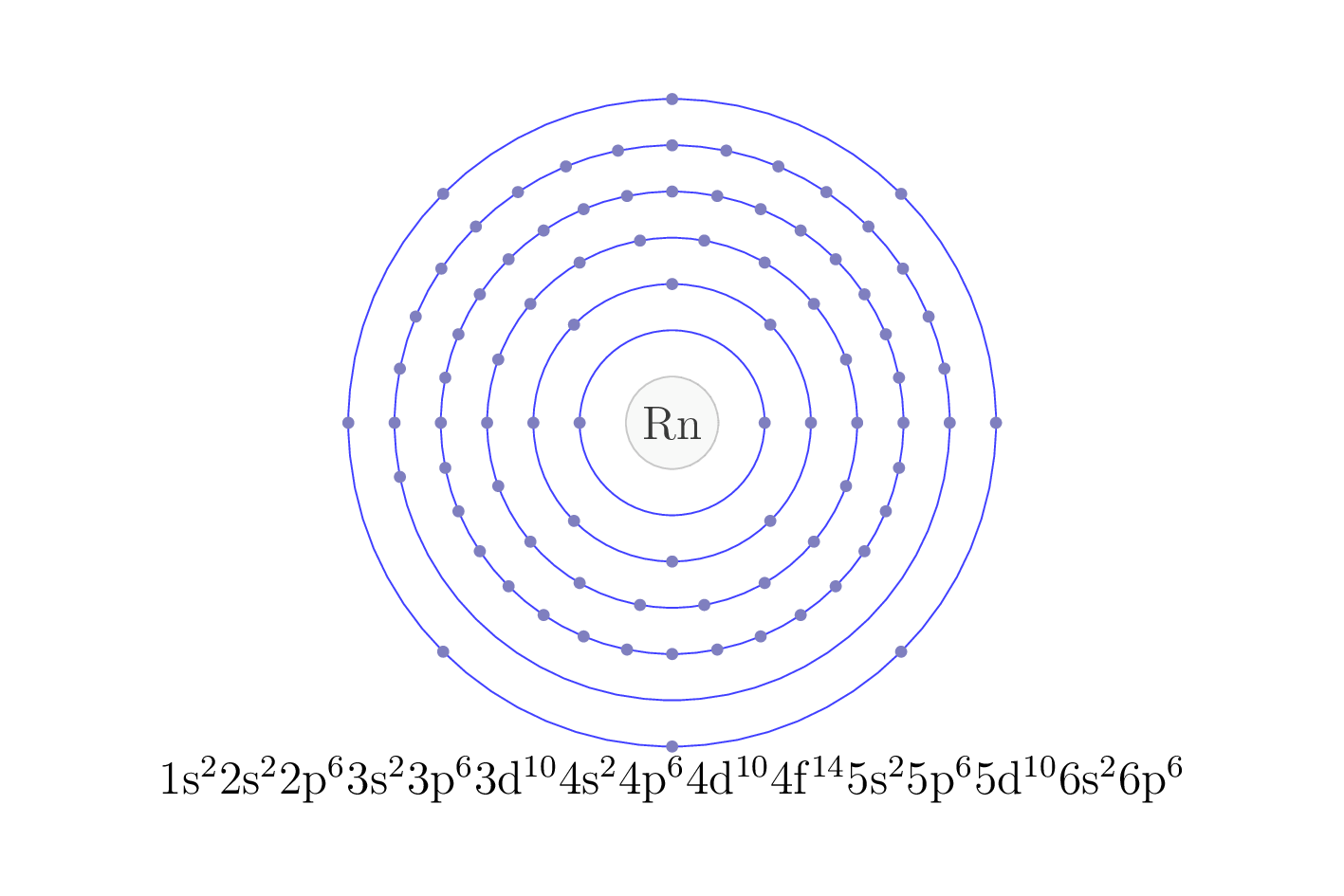## Electron configuration

The electron configuration of an element describes the arrangement of electrons in the atoms of that element, and be used to predict its chemical properties and reactivity.

In the electron configuration notation, the letters "s", "p", "d", and "f" represent the different types of atomic orbitals, and the superscripts indicate the number of electrons in each orbital. The orbitals are filled in a specific order, starting with the lowest energy orbital and working up.## Emission spectra

Each element in the periodic table presents its own unique emission spectra, which is determined by the energy levels of its electrons. When an electron in an atom is excited to a higher energy level, it can de-excite by emitting a photon of light with an energy equal to the difference between the two levels. This results in a characteristic emission line in the spectra (which corresponds to specific wavelengths of light). These spectra are usefull to identify the elements present in a sample.## Symmary of properties (Rn)

 Atomic weight  Discoverer (year) Dorn, Friedrich Ernst (1900) Natural form gas Electron configuration [Xe] 4f 14 5d10 6s2 6p6 M.p. (ºC) -71 B.p. (ºC) -62 Earth's crust abundance (ppm) <0.001 Isotope (abundance %) Density (g/cm3) 9.73 vdW radius (pm) 220 Covalent radius (pm) 146 Electronegativity (Pauling) Vaporisation enthalpy (Kj/mol) 18.10 Fusion enthalpy (kJ/mol) 2.89 Specific heat capacity (J/g·K) at 25ºC and 1 at 0.09 Thermal conductivity (W/cm·K) at 25 ºC and 1 at <0.001 Oxidation number 0 Electronic affinity (eV) unstable ion 1st Ionization energy (eV) 10.7485

#### Definition of terms in the previous table

• Atomic weight: The average mass of an element's atoms, typically given in atomic mass units (amu).
• Natural form: The most stable and abundant form of an element that occurs naturally in the environment.
• Electron configuration: The arrangement of electrons in an atom or molecule.
• Melting point: The temperature at which a solid substance turns into a liquid.
• Boiling point: The temperature at which a liquid substance turns into a gas.
• Earth's crust abundance (ppm): The concentration of an element in the Earth's crust, typically given in parts per million (ppm).
• Isotope (abundance %): A variant of an element that has the same number of protons in the nucleus, but a different number of neutrons. The abundance of an isotope is the percentage of the isotope in a sample of the element.
• Density (g/cm3): The mass of a substance per unit volume.
• vdW radius (pm): The radius of an atom or molecule as predicted by the van der Waals model, typically given in picometers (pm).
• Covalent radius (pm): The distance from the center of an atom to the center of another atom with which it is bonded covalently, typically given in picometers (pm).
• Electronegativity (Pauling): A measure of an atom's ability to attract electrons in a chemical bond, based on the Pauling scale.
• Vaporisation enthalpy (kJ/mol): The amount of energy required to convert a substance from a liquid to a gas at a constant temperature.
• Fusion enthalpy (kJ/mol): The amount of energy required to convert a substance from a solid to a liquid at a constant temperature.
• Specific heat capacity (J/g·K) at 25ºC and 1 at: The amount of heat required to raise the temperature of 1 gram of a substance by 1 degree Celsius at a constant pressure.
• Thermal conductivity (W/cm·K) at 25 ºC and 1 at: The ability of a substance to conduct heat, typically given in watts per centimeter per kelvin.
• Oxidation number: A positive or negative integer that represents the number of electrons that an atom has gained or lost in a chemical compound.
• Electronic affinity: The energy change associated with adding an electron to a neutral atom to form a negative ion.
• 1st Ionization energy: The energy required to remove the most loosely bound electron from a neutral atom.

Back to the Periodic Table of the Elements.

Shares